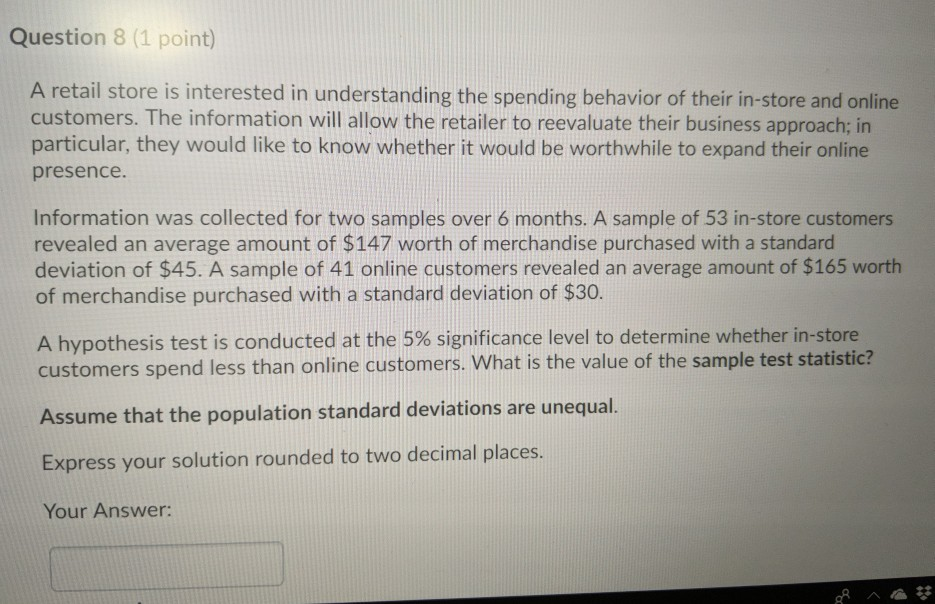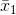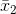# Question 8 (1 point) A retail store is interested in understanding the spending behavior of their...Question 8 (1 point) A retail store is interested in understanding the spending behavior of their in-store and online customers. The information will allow the retailer to reevaluate their business approach; in particular, they would like to know whether it would be worthwhile to expand their online presence. Information was collected for two samples over 6 months. A sample of 53 in-store customers revealed an average amount of $147 worth of merchandise purchased with a standard deviation of$45. A sample of 41 online customers revealed an average amount of $165 worth of merchandise purchased with a standard deviation of$30. A hypothesis test is conducted at the 5% significance level to determine whether in-store customers spend less than online customers. What is the value of the sample test statistic? Assume that the population standard deviations are unequal. Express your solution rounded to two decimal places Your Answer:

For Instore customers:= $147, s1 =$45, n1 = 53

For Online Customers:= $165, s2 =$30, n2 = 41

Since we assume unequal variances

The Test Statistic is given by##### Add Answer of: Question 8 (1 point) A retail store is interested in understanding the spending behavior of their...
More Homework Help Questions Additional questions in this topic.